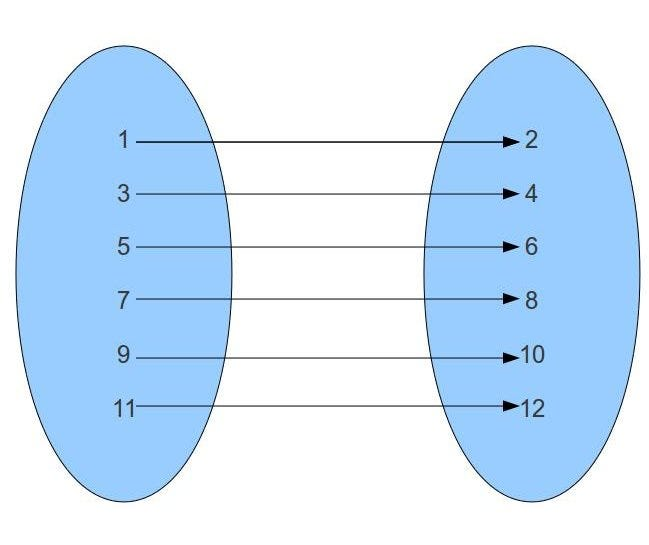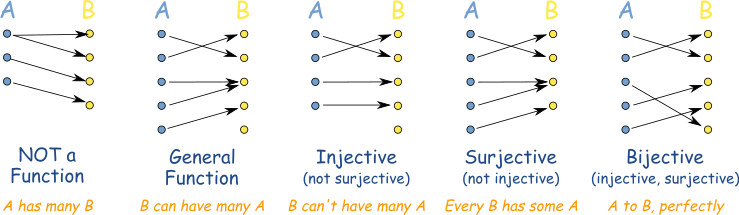#### Flashcards#

I suggest making your own flashcards to study, but if you don’t want to you can use my flashcard deck here.

A function is just like a function in programming. It takes an input, performs some maths on it, then outputs the result.

The syntax of a function is f(x) = 2 * x. This means given x, the function will return 2*x.

A function cannot have more than one output if given a single input. However, multiple inputs can lead to the same output.

Functions can be written in two ways, either f(x) = 2 * x or f: R→ Rwhich defines the input and output to be real numbers.

#### Domain, Codomain, Range#

Let function f(x) = 2x. The set, x, is the Domain; the range of all possible inputs. The set 2x is the codomain. The values produced by the function is the range.

In other syntax, f: A → B. A is called the domain, B is the **co-domain **and the **range **of f(A) is F(A) = {f(x) | x ∈ A}

#### Finding the domain of a function#

It is best to show an example of how to find the domain of a function.

f(x) = sqrt(2x-8) We know that the domain of a function is the set of all possible inputs into a function. We know that the above function is only defined when it’s taking the square root of a non-negative number so it’s only going to be defined when: 2x-8 ≥ 0. Now this is a simple algebraic problem to find x. 2x ≥ 8 x ≥ 4So the domain here is the set of all real numbers ≥ 4.

Sometimes it is easier to find what cannot be in the domain than to find every item in the domain.

Given the function f(x) = 2/x-3, let’s try find the range.

Is there anything that X CANNOT be? Yes! If X is 0 it would cause an error as you cannot divide by 0!

#### Types of functions#

Label the left hand set A and the right hand set B and ignore the lines.#### A has many B#

Not a function, because function inputs cannot map to more than one output.

#### B can have many A#

It is a function because a single output can be produced from different inputs.

#### B can’t have many A (Injective)#

Not all outputs of B can be produced by A.

#### Every B has some A (Surjective)#

Every output in B has at least one input in A, sometimes more.

#### A to B, perfectly (Bijective)#

Bijective is the combination of injective and surjective. Every A matches perfectly to an output.https://www.mathsisfun.com/sets/injective-surjective-bijective.html

#### Cardinality of sets#

The cardinality of a set is how many items are in the set, denoted as |a|

#### Powersets#

The powerset is the set containing every single subset of set A, where A is any set. The powerset of a = {1, 2, 3} is:

Pow(A)= { {1}, {2}, {3}, {1,2}, {1,3}, {2, 3}, {1, 2, 3}, {Ø} }

The cardinality of a power set is always 2^n where n is |a|. The same cardinality rule applies to bit vector sets.

#### Infinite sets#

Infinite sets can also be bijective, injective or surjective.

#### Uncountable sets#

There exists sets that are not countable, such as Cantor’s set derived from Cantor’s diagonal argument.

#### The Pigeon Hole Principle#

The Pigeon Hole Principle states that if |A| > |B| then at least one value of F occurs more than once.

In other words, if there are N holes and we have N+1 pigeon then 2 pigeons must occupy the same hole.